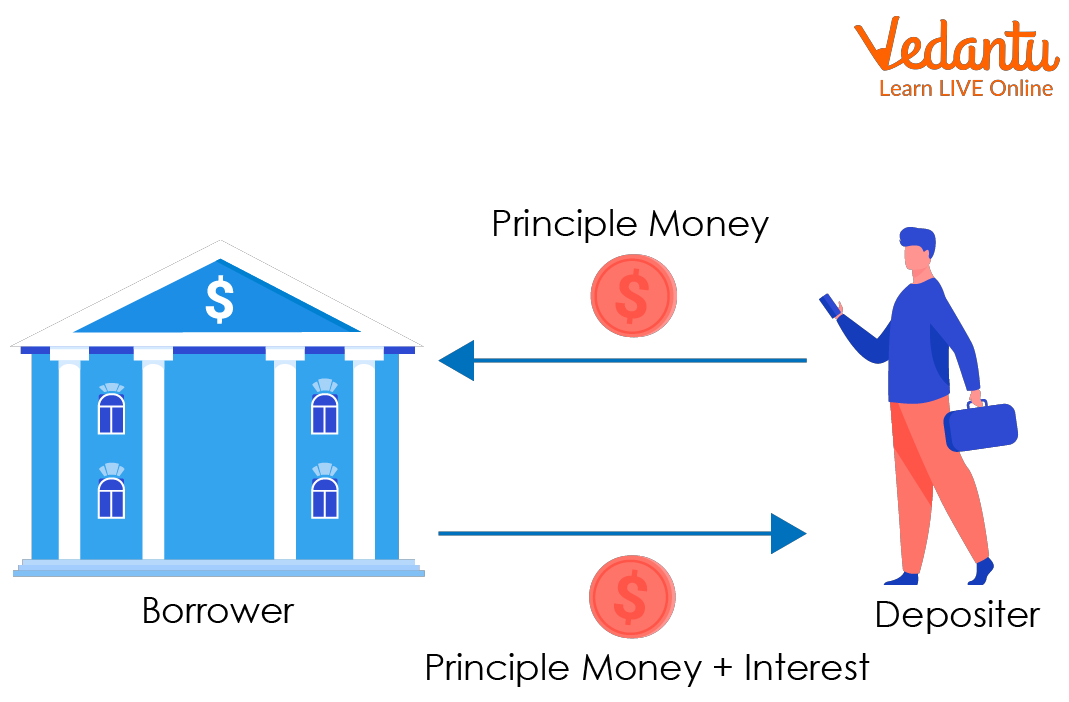Courses
Courses for Kids
Free study material
Offline Centres
More

# Formula to Calculate Simple InterestLast updated date: 28th Nov 2023
Total views: 61.8k
Views today: 1.61kWhen one deposits money in a bank, the bank applies to interest on the money deposited. Banks use various types of interest, and one of them is simple interest. Simple Interest is a quick and easy way to calculate the interest on your money, where interest is always applied to the principal at the same rate for each time period. Simple interest is determined by multiplying the principal by the daily interest rate by the number of days elapsed between payments.

### What is Simple Interest?(Simple Interest Definition)

• Interest is the income you receive from deposits or fees you pay on loans.

• Simple Interest is a measure of interest that does not account for interest or accruals over multiple periods. The interest rate applies only to the principal of the loan or investment and is not affected by interest accrued.Simple Interest

### How to Find Simple Interest?

Simple Interest is calculated by using the formula:

${\rm{SI}} = {\rm{P}} \times {\rm{R}} \times {\rm{T}}$

Where,

P = Principal

R=Percentage rate of Interest per annum [year]

T=time taken in the yearSimple Interest Formula Example

### Terms Related to Simple Interest

Some terms like Principal, rate and time used to find Simple Interest. Let’s discuss each:

• Principal: The amount borrowed from the bank by a person is called the Principal. The head is designated by the letter P.

• Rate: The interest rate is the interest rate at which the principal is paid to someone over a period of time, which could be 5% or 15% etc. The interest rate  is denoted by R.

• Time: Time is a period given to someone on the principal amount. Time is indicated by the letter T.

• Amount: When you get a loan from a bank, you must repay the principal plus interest, and this repayment is called the amount. So, a sum of money at simple interest amounts to the money you have to pay back. The amount is denoted by A.

Thus ${\rm{A = P + I}}$

## Conclusion

On a specific principal, simple interest remains constant year after year. Consider the day you must return the money, not the day you borrowed it, to determine the amount of time. The interest rate is always given as a fraction in the formula.

### Solved Examples

1. If the principal is Rs. 2500 for 1 year at the rate of 15%.Calculate simple interest.

Solution: Using the  simple interest formula

${\rm{SI}} = {\rm{P}} \times {\rm{R}} \times {\rm{T}}$

Here  P $=$2500, T $=$1year and ${\rm{R}} = 15\% \left[ {\frac{{15}}{{100}}} \right]$

So in the above value

${\rm{SI}} = 2500 \times \frac{{15}}{{100}} \times 1$

${\rm{SI}} = 375$

So,  simple interest is  375.

2.Mohan pays back 7,000 rupees equal to 5,000 rupees borrowed over two years. Find the interest rate.

Solution: A $=$7000rupees

P $=$5000rupees

We know ${\rm{A}} = {\rm{SI}} + {\rm{P}}$

${\rm{SI}} = {\rm{A}} - {\rm{P}}$

${\rm{SI}} = {\rm{A}} - {\rm{P}} = 7000 - 5000 = 2000$ rupees

T $=$ 2years

Also

${\rm{SI}} = \frac{{[{\rm{P}} \times {\rm{R}} \times {\rm{T}}]}}{{100}}$

${\rm{R}} = \frac{{100{\rm{SI}}}}{{{\rm{P}} \times {\rm{T}}}}$

${\rm{R}} = \frac{{[100 \times 2000]}}{{[5000 \times 2]}}$

${\rm{R}} = 20\%$

So ,${\rm{R}} = 20\%$

3. Naman borrowed Rs 20,000 for 3 years at an interest rate of 3% per annum. Find of the accumulated interest at the end of 3 years.

Solution:   P $=$20,000rupees

${\rm{R}} = 3\%$

T $=$3years

${\rm{SI}} = \frac{{[{\rm{P}} \times {\rm{R}} \times {\rm{T}}]}}{{100}}$

${\rm{SI}} = \frac{{[20000 \times 3 \times 3]}}{{100}}$

${\rm{SI}} = 1800$

Therefore accumulated interest at the end of 3 years is 1800

## FAQs on Formula to Calculate Simple Interest

1. Give some real-life examples of  Simple Interest.

Simple Interest is a quick and easy way to calculate the interest on your money, where interest is always applied to the principal at the same rate for each time period. Some real-life examples we have seen in our daily lives are Car loans, Home loans, and Education loans.

2. Why is simple interest easy to calculate.

The simple interest is easy to calculate as it is calculated only on the base principal amount, not principal and interest.

3. How does simple interest change with the principal?

Simple Interest remains constant on principal.

4. Difference between interest and simple interest.

Interest is the income you receive from deposits or fees you pay on loans.

Simple interest is a measure of interest that does not account for interest or accruals over multiple periods.